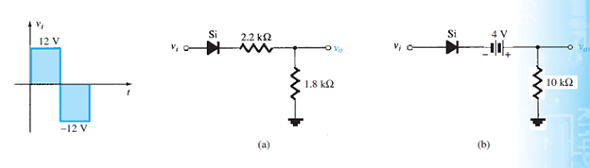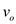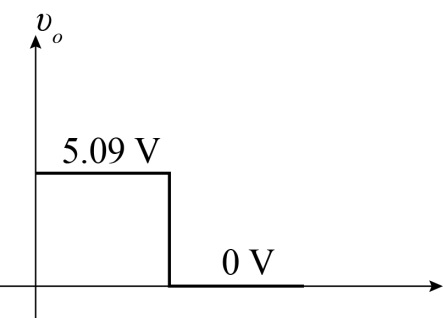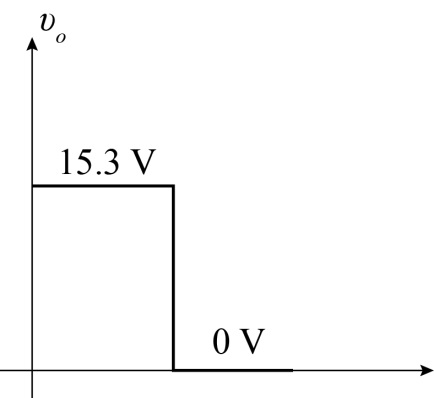Problem

# Determine vo for each network of Fig. 2.177 for the input shown.FIG. 2.177

Determine vo for each network of Fig. 2.177 for the input shown.

FIG. 2.177#### Step-by-Step Solution

Solution 1

(a)

Refer to the circuit diagram shown in Fig. $$2.176$$ (a) in the textbook.

As per Fig. $$2.176$$ (a) for the positive pulse of input voltage $$v_{i}$$ the expression for output voltage

$$v_{o}$$ is,

$$v_{o}=\frac{R_{2}\left(V-V_{D}\right)}{R_{2}+R_{1}}$$

Here,

Resistance $$R_{1}$$ is $$2.2 \mathrm{k} \Omega$$

Resistance $$R_{2}$$ is $$1.8 \mathrm{k} \Omega$$

Voltage $$V$$ is $$12 \mathrm{~V}$$

Silicon Diode voltage $$V_{D}$$ is $$0.7 \mathrm{~V}$$

Substitute the corresponding values to obtain $$v_{o}$$ as follows:

\begin{aligned} v_{o} &=\frac{R_{2}\left(V-V_{D}\right)}{R_{2}+R_{1}} \\ &=\frac{1.8 \mathrm{k} \Omega(12 \mathrm{~V}-0.7 \mathrm{~V})}{1.8 \mathrm{k} \Omega+2.2 \mathrm{k} \Omega} \\ &=5.09 \mathrm{~V} \end{aligned}

Hence, for the positive pulse the output voltage $$v_{o}$$ is $$5.09 \mathrm{~V}$$.

As per Fig. $$2.176$$ (a) for the negative pulse of input voltage $$v_{i}$$ the output voltage $$v_{o}$$ when the

diode is open is given as:

$$v_{o}=0 \mathrm{~V}$$

Hence, for the negative pulse the output voltage $$v_{o}$$ is $$0 \mathrm{~V}$$.

The output voltage waveformis given as:Figure 1

(b)

Refer to the circuit diagram shown in Fig. $$2.176$$ (b) in the textbook.

As per Fig. $$2.176$$ (b) for the positive pulse of input voltage $$v_{i}$$ the expression for output voltage $$v_{o}$$ is,

$$v_{o}=V-V_{D}+E$$

Here,

Voltage $$V$$ is $$12 \mathrm{~V}$$

Silicon Diode voltage $$V_{D}$$ is $$0.7 \mathrm{~V}$$

Battery supply $$E$$ is $$4 \mathrm{~V}$$

Substitute the corresponding values to obtain $$v_{o}$$.

\begin{aligned} v_{o} &=V-V_{D}+E \\ &=12 \mathrm{~V}-0.7 \mathrm{~V}+4 \mathrm{~V} \\ &=15.3 \mathrm{~V} \end{aligned}

Hence, for the positive pulse the output voltage $$v_{o}$$ is $$15.3 \mathrm{~V}$$.

As per Fig. $$2.176$$ (b) for the negative pulse of input voltage $$v_{i}$$ the output voltage $$v_{o}$$ when the

diode is open is given as:

$$v_{o}=0 \mathrm{~V}$$

Hence, for the negative pulse the output voltage $$v_{o}$$ is $$0 \mathrm{~V}$$.

The output voltage waveformis given as:Figure 2